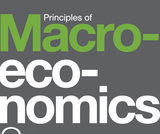# 8 Results

View
Selected filters:
• SpecializationUnrestricted Use
CC BY
Rating

This course describes discrete mathematics, which involves processes that consist of sequences of individual steps (as compared to calculus, which describes processes that change in a continuous manner). The principal topics presented in this course are logic and proof, induction and recursion, discrete probability, and finite state machines. Upon successful completion of this course, the student will be able to: Create compound statements, expressed in mathematical symbols or in English, to determine the truth or falseness of compound statements and to use the rules of inference to prove a conclusion statement from hypothesis statements by applying the rules of propositional and predicate calculus logic; Prove mathematical statements involving numbers by applying various proof methods, which are based on the rules of inference from logic; Prove the validity of sequences and series and the correctness or repeated processes by applying mathematical induction; Define and identify the terms, rules, and properties of set theory and use these as tools to support problem solving and reasoning in applications of logic, functions, number theory, sequences, counting, probability, trees and graphs, and automata; Calculate probabilities and apply counting rules; Solve recursive problems by applying knowledge of recursive sequences; Create graphs and trees to represent and help prove or disprove statements, make decisions or select from alternative choices to calculate probabilities, to document derivation steps, or to solve problems; Construct and analyze finite state automata, formal languages, and regular expressions. (Computer Science 202)

Subject:
Computer Science
Material Type:
Full Course
Provider:
The Saylor Foundation
04/29/2019Unrestricted Use
CC BY
Rating

Principles of Macroeconomics 2e covers the scope and sequence of most introductory economics courses. The text includes many current examples, which are handled in a politically equitable way. The outcome is a balanced approach to the theory and application of economics concepts. The second edition has been thoroughly revised to increase clarity, update data and current event impacts, and incorporate the feedback from many reviewers and adopters. Changes made in Principles of Macroeconomics 2e are described in the preface and the transition guide to help instructors transition to the second edition. The first edition of Principles of Macroeconomics by OpenStax is available in web view here.

Subject:
Economics
Material Type:
Full Course
Provider:
Rice University
Provider Set:
OpenStax College
06/29/2017Unrestricted Use
CC BY
Rating

By the end of this section, you will be able to:

Explain the gains of trade created when a country specializes

Subject:
Applied Science
Material Type:
Module
Provider:
Rice University
Provider Set:
OpenStax College
09/20/2018Unrestricted Use
CC BY
Rating

Subject:
Applied Science
Material Type:
Module
Provider:
Rice University
Provider Set:
OpenStax College
09/20/2018Unrestricted Use
CC BY
Rating

Subject:
Applied Science
Material Type:
Module
Provider:
Rice University
Provider Set:
OpenStax College
09/20/2018Unrestricted Use
CC BY
Rating

By the end of this section, you will be able to:

Discuss the importance of studying economics
Explain the relationship between production and division of labor
Evaluate the significance of scarcity

Subject:
Applied Science
Material Type:
Module
Provider:
Rice University
Provider Set:
OpenStax College